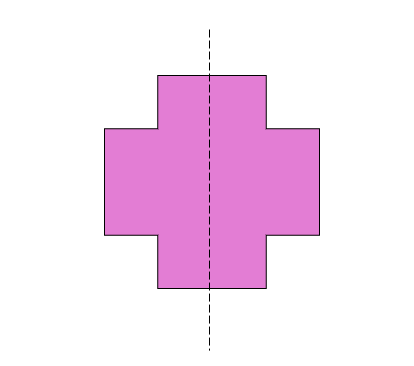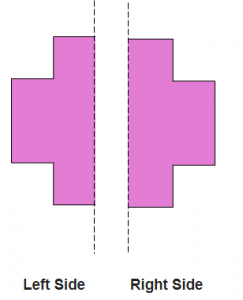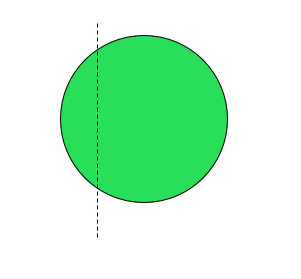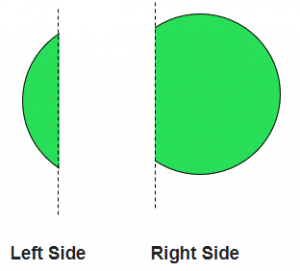# What is Symmetry | Symmetry Examples

What is Symmetry?

A figure is said to be symmetrical about the dotted line, if on folding, one part will fit exactly over the other part.

### Symmetry Examples

Example 1

Is the given figure is symmetrical about the dotted line?Explanation:

A figure is said to be symmetrical about the dotted line, if on folding, one part will fit exactly over the other part.

If we separate the given image on the dotted line, it would be like thisSince the image on the left side of the dotted line is same in shape from the image on the right side of the dotted line, although its different in appearance, on folding left side image would exactly fit over the right side image. Hence, we can say that the given figure is symmetrical about dotted line.

Example 2

Is the given figure, symmetrical about the dotted line?Explanation:

A figure is said to be symmetrical about the dotted line, if on folding, one part will fit exactly over the other part.

If we separate the given image on the dotted line, it would be like thisSince the image on the left side of the dotted line is different in shape from the image on the right side of the dotted line, on folding, left side image would not exactly over the right side image. Hence, we can say that the given figure is not symmetrical about dotted line.